# High School Algebra - Graphing and Factoring Quadratic Equations: Tutoring Solution Chapter Exam

Exam Instructions:

Choose your answers to the questions and click 'Next' to see the next set of questions. You can skip questions if you would like and come back to them later with the yellow "Go To First Skipped Question" button. When you have completed the practice exam, a green submit button will appear. Click it to see your results. Good luck!

### Page 1

#### Question 1 1. If the equation below told you a ball was 'h' feet in the air after 't' seconds, what would be the first step to finding out when it hit the ground?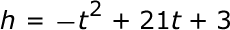#### Question 3 3. When completing the square for the quadratic equation shown below, how is c calculated?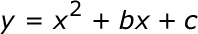### Page 2

#### Question 10 10. What kind of graph is shown?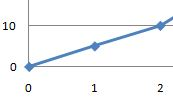### Page 3

#### Question 13 13. Factor and solve.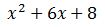#### Question 15 15. In order to complete the square on the following equation, what value would you add to both sides?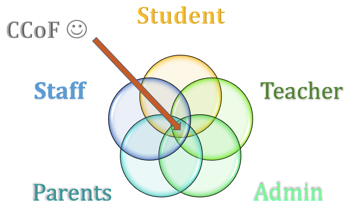### Page 4

#### Question 18 18. Factor and solve.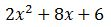### Page 5

#### Question 24 24. Rewrite the following equation in vertex form.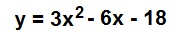### Page 6

#### Question 29 29. Factor and solve.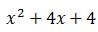#### High School Algebra - Graphing and Factoring Quadratic Equations: Tutoring Solution Chapter Exam Instructions

Choose your answers to the questions and click 'Next' to see the next set of questions. You can skip questions if you would like and come back to them later with the yellow "Go To First Skipped Question" button. When you have completed the practice exam, a green submit button will appear. Click it to see your results. Good luck!

Support